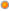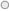# Data Analysis Expressions (DAX) In PowerPivot for Excel 2010

User Rating: 4 / 5B. Executive Summary 3
C. Background 4
1. PowerPivot 4
2. PowerPivot for Excel 5
3. Samples – Contoso Database 8
D. Data Analysis Expressions (DAX) – The Basics 9
1. DAX Goals 9
2. DAX Calculations - Calculated Columns and Measures 9
3. DAX Syntax 13
4. DAX uses PowerPivot data types 13
5. Intro to Context – Row Context and Filter Context 14
6. Functionality that doesn’t exist in Excel formulas 15
7. DAX Operators and Constants 16
E. Simple DAX Functions 16
1. BLANK() and Blank values 17
2. Functions from Excel 17
3. FORMAT (Value, Format_text) 18
4. Functions to aggregate expressions – the “X” functions 19
5. COUNTROWS (Table) 20
6. RELATED (Column) and RELATEDTABLE (Table) 20
7. FILTER(Table, Condition) and DISTINCT (Column) 22
F. Row Context and Filter Context 24
1. Row Context 24
2. Filter Context 26
3. Relationships and Filter Context 27
4. Measures and Filter Context 27
G. More DAX Functions 28
1. CALCULATE(Expression, SetFilter1, SetFilter2,...) 28
2. VALUES(column) 32
3. CALCULATETABLE(TableExpression, SetFilter1, SetFilter2,...) 33
4. ALL(Table) and ALL(Column1 [,Column2]...) 33
5. ALLEXCEPT (Table [,Column1] [,Column2]...) 36
H. Time Intelligence Functions 36
1. Concepts and Best Practices 36
2. Functions that return a single date 38
3. Functions that return a table of dates 42
Year over Year Growth 43
Calculating many time periods within a single measure formula 44
4. Functions that evaluate expressions over a time period 49
I. Sample Formulas 50
1. Calculated Columns 50
2. Measures 50

### B. Executive Summary

There are millions of Microsoft Excel users who are familiar with using Excel formulas to perform calculations.  Those calculations may be as simple as adding up a column of numbers, or they may be far more complex simulations of various business models.  But in every case, each formula is built using a combination of basic operators and functions that are provided within Excel as the building blocks for such formulas.

PowerPivot for Excel provides the building blocks needed to build business intelligence solutions, whether those solutions use simple calculations or something significantly more complex.  The building blocks include the ability to import data tables from a wide variety of data sources, the ability to perform calculations on large volumes of in-memory data quickly, the ability to author custom calculations using the DAX (Data Analysis Expressions) language, and the ability to use the result of those calculations in Excel PivotTables.

Data Analysis Expressions are very similar to Excel formulas, and there is considerable overlap between the list of DAX functions and Excel functions.  But there are significant differences, and many new functions in DAX that don’t exist within Excel.  These functions are designed to offer capabilities that focus on data analysis, particularly for related tables of data, and for dynamic analysis.  The ability to define calculations that will be evaluated dynamically in many different contexts is a powerful tool, and prior to PowerPivot and DAX, these sorts of calculations often involved more complex multi-dimensional concepts and languages.
With Data Analysis Expressions, it is our hope that Excel users will be able to easily learn how to perform data analysis, using DAX formulas that look a lot like Excel formulas, but that provide additional capabilities, and that are much easier to learn and use than the multi-dimensional constructs more generally used by IT professionals to perform this sort of data analysis.

This paper outlines the use of DAX formulas in PowerPivot, and describes the many new DAX functions.  In addition to covering the functions themselves, there is a discussion of the important concepts that any PowerPivot user will want to know.  It is hoped that this paper might be a good way to become familiar with the basics of the DAX formula language.